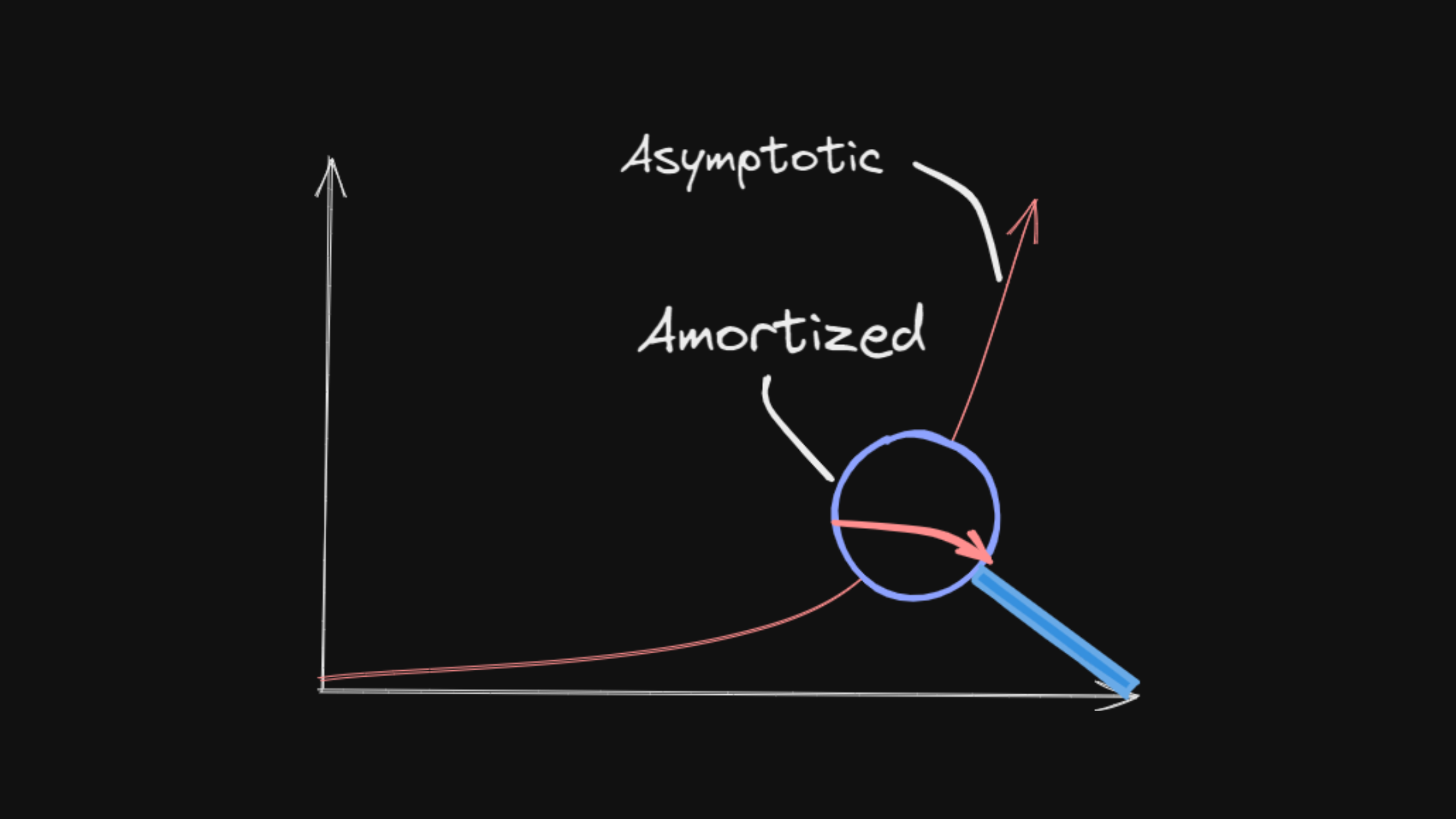Yash Singh# Amortization

While preparing for the USACO Contest, I learned several techniques for amortized analysis of algorithms. In this article I will share a few of them.

## Subsets over Subsets

When iterating over the subsets over each subset, the time complexity is $\mathcal{O}(3^n)$. This is because the time complexity is $\sum_{k=0}^{n} 2^k\cdot\binom{n}{k}=(1+2)^n=3^n$ because if we expand this out with the binomial theorem, it will represent the cost of every subset.

## Two Pointers

The two pointers approach involves maintaining two pointers and shifting them along throughout the array. Even though it is implemented as a double loop, which would look like a $\mathcal{O}(n^2)$, however we notice that each element is visited only twice. An example code snippet of this would look like:

int r{0};
for (int l{0}; l < n; ++l) {
while (r < n && a[r] < a[l]) ++r;
cout << r-l+1 << "\n";
}

In this code snippet we notice that each node is visited at most once by the r pointer and the l pointer.

## Maximum Nodes/Edges

One other amortization technique that can be used inside programming contests is the fact that given there are $N$ nodes and $M$ edges, there are at most $\sqrt{M}$ nodes and at most $N^2$ edges. A great example of using this inside a problem is the second official solution to USACO December 2022 Gold Problem 3, Strongest Friendship Groups.

## DSU Amortized

Another example of amortization is the DSU data structure. This data structure shows how we can make queries and updates extremely efficient in DSU. For those who are interested there are several proofs for why the $\mathcal{O}(\alpha(n))$ and $\mathcal{O}(\log^*n)$ algorithms works.

## Techniques for finding

When identifying the amortized complexity here are a few techniques to use:

• Identify the search space -- Identify what you are searching through, its size, etc. This will help you find places for improvement and the actual complexity of your program.
• Repetition -- Look for spots of repetition. Whenever there is repetition, then you can cache values or results. One example problem that uses Number Theory to look for repetition is USACO Silver - Loan Repayment. Try to find the repetition in the results and look for a faster algorithm to solve it.
• Expected Value -- Sometimes your program is faster than it seams because at the high peak of operations only happens in edge cases which in fact makes another part of your program faster. When evaluating the complexity of your program, try identifying a probability distribution and find the expected value of operations. Note however that this only comes in handy if you are going to search through every state in the search space, because judges often design their test cases to make your program run slower.
• Don't analyze it -- Many times if you feel like you have some idea that would asymptotically seem slow, but might be faster when run amortized, just write it out and submit it to the judge. Most competitive programming contests don't give you the time to write out a formal proof for your complexity.